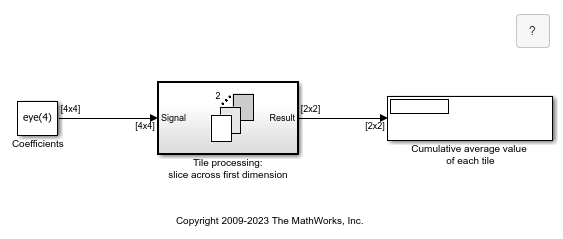Tiled Processing of 2D Signals with For Each Subsystem

This example shows how to use the For Each Subsystem. In this example the operations are performed on matrices.

Open the example model sldemo_foreach_tiled. This model contains a For Each subsystem that processes submatrices of a matrix input signal.

To process submatrices of a matrix signal, you will need to use nested For Each subsystems. The outer subsystem can be used to partition the signal along the first dimension, while the inner one can be used to partition along the second dimension. This order is arbitrary, and can be changed without affecting the result.

In this example a 4-by-4 matrix is to be partitioned into 2-by-2 submatrices. The outer subsystem partitions the signal along the first dimension. The resulting subarrays are then partitioned by the inner subsystem along the second dimension. Concatenation of the outputs respects the partitioning order to preserve the shape of the input signal. Note, however, that the output dimensions change since the processing of the submatrices results in scalar outputs.

To see a tile repetition implementation for this model, double-click on the subsystem in the lower-right corner of the model window. This implementation does not use the For Each Subsystem. Instead, it mimics the functionality of the For Each Subsystem by replicating the subsystem as many times as necessary and by selecting and concatenating the signals appropriately. This replication process is prone to error and is not scalable -- changing the input signal size would require changing the model accordingly.